• 5.28 多元函数 聚点：去心邻域（四面八方趋近 值相等 -> 极限才存在） 二阶偏导在连续条件下与求导次序无关 二元函数的极值 必要条件：一阶偏导求驻点 充分条件：二阶偏导判存在 条件极值 拉格朗日乘数法 局限：...
5.28 多元函数
聚点：去心邻域（四面八方趋近 值相等 -> 极限才存在）
二阶偏导在连续条件下与求导次序无关
二元函数的极值
必要条件：一阶偏导求驻点
充分条件：二阶偏导判存在
条件极值
拉格朗日乘数法

局限：

方程组求解困难
只是必要条件，而非充要条件
多变量情况下，需根据二阶偏导数构成的海森矩阵的正定性判断是否属于极值点

二元函数的全微分
切平面近似表示
方向导数 & 梯度
偏导数 --任意方向变化率–> 方向导数（用于搜索函数极值，找最陡的【局部最优解】）
射线的方向方程：
单位方向向量
梯度：grad f(x0, y0) 是一个向量
指示了函数变化率最大的方向
如何按梯度方向移动？
next_x = x0 + t * fx(x0, y0)
next_y = y0 + t * fy(x0, y0)
梯度下降算法
迭代关系式：
极大值迭代式：
极小值迭代式：
step 1: 初始化
m: 迭代步长 / 学习率 （选取和函数特性有关，参数）

case: gradient_descent

# 用梯度下降算法求函数最小值：L = x1 ^ 2 + 2 * x2 ^ 2

# 作图
import matplotlib.pyplot as plt

m = 0.1
x1 = 10
x2 = 3
L = x1 ** 2 + 2 * x2 ** 2 # grad L = (2 * x1, 4 * x2)
times = 0
err = 1
threshold = 0.0000001
value = []

while (err > threshold and times < 10000):
x1 = x1 - m * (2 * x1)
x2 = x2 - m * (4 * x2)
err = abs(x1 ** 2 + 2 * x2 ** 2 - L)    # 易出错：计算前后两次迭代后函数差值的绝对值
value.append(err)
L = x1 ** 2 + 2 * x2 ** 2
times += 1

print(x1, x2, L, times)
# 作图
plt.plot(value)
plt.show()

当有不同极值点时，可能收敛到不同值

局限性：

初值敏感，变量越多越敏感，不同初值可能收敛到不同极值点
学习率敏感，可动态改变学习率
无法完全保证取得全局最值


展开全文• 1.一元函数与多元函数复合的情形 定理1：如果函数u=φ(t)u=\varphi (t)u=φ(t)及v=ψ(t)v=\psi(t)v=ψ(t)都在点t可导，函数z=f(u,v)z=f(u,v)z=f(u,v)在对应点(u,v)(u,v)(u,v)具有连续偏导数，那么复合函数z=f[φ(t),...
多元复合函数不同的复合形式，分三种情形讨论。
1.一元函数与多元函数复合的情形
定理1：如果函数$u=\varphi (t)$及$v=\psi(t)$都在点t可导，函数$z=f(u,v)$在对应点$(u,v)$具有连续偏导数，那么复合函数$z=f[\varphi(t),\psi(t)]$在点t可导，且有
$\frac{dz}{dt}=\frac{\partial z}{\partial u}\frac{du}{dt}+\frac{\partial z}{\partial v}\frac{dv}{dt} \tag{1}$
用同样的方法，可把定理推广到复合函数的中间变量多于两个的情形。
例如，设
$z=f(u,v,w),u=\varphi(t),v=\psi(t),w=w(t)$
复合而得复合函数
$z=f[\varphi(t),\psi(t),w(t)]$
则在与定理相类似的条件下，这复合函数在点t可导，且其导数可用下列公式计算：
$\frac{dz}{dt}=\frac{\partial z}{\partial u}\frac{du}{dt}+\frac{\partial z}{\partial v}\frac{dv}{dt}+\frac{\partial z}{\partial w}\frac{dw}{dt} \tag{2}$
在公式（1）及公式（2）中的导数$\frac{dz}{dt}$称为全导数。
2. 多元函数与多元函数复合的情形
定理2：如果函数$u=\varphi(x,y)$及$v=\psi(x,y)$都在点$(x,y)$具有对x及对y的偏导数，函数$z=f(u,v)$在对应点$(u,v)$具有连续偏导数，那么复合函数$z=f[\varphi(x,y),\psi(x,y)]$在点$(x,y)$的两个偏导数都存在，且有
$\frac{\partial z}{\partial x}=\frac{\partial z}{\partial u}\frac{\partial u}{\partial x}+\frac{\partial z}{\partial v}\frac{\partial v}{\partial x} \\ \frac{\partial z}{\partial y}=\frac{\partial z}{\partial u}\frac{\partial u}{\partial y}+\frac{\partial z}{\partial v}\frac{\partial v}{\partial y}$
类似地，设$u=\varphi(x,y)、v=\psi(x,y)$及$w=\omega(x,y)$都在点$(x,y)$具有对x及对y的偏导数，函数$z=f(u,v,m)$在对应点$(u,v,w)$具有连续偏导数，则复合函数
$z=f([\varphi(x,y),\psi(x,y),\omega(x,y)])$
在点$(x,y)$的两个偏导数都存在，且可用下列公式计算：
$\frac{\partial z}{\partial x}=\frac{\partial z}{\partial u}\frac{\partial u}{\partial x}+\frac{\partial z}{\partial v}\frac{\partial v}{\partial x}+\frac{\partial z}{\partial w}\frac{\partial w}{\partial x} \\ \frac{\partial z}{\partial y}=\frac{\partial z}{\partial u}\frac{\partial u}{\partial y}+\frac{\partial z}{\partial v}\frac{\partial v}{\partial y}+\frac{\partial z}{\partial w}\frac{\partial w}{\partial y}$
3.其他情形
定理3：如果函数$u=\varphi(x,y)$在点$(x,y)$具有对x及对y的偏导数，函数$v=\psi(y)$在点y可导，函数$z=f(u,v)$在对应点$(u,v)$具有连续偏导数，那么复合函数$z=f[\varphi(x,y),\psi(y)]$在点$(x,y)$的两个偏导数都存在，且有
$\frac{\partial z}{\partial x}=\frac{\partial z}{\partial u}\frac{\partial u}{\partial x} \\ \frac{\partial z}{\partial y}=\frac{\partial z}{\partial u}\frac{\partial u}{\partial y}+\frac{\partial z}{\partial v}\frac{dv}{dy}$
上述情形实际上是情形2的一种特例，即在情形2中，如变量v与x无关，从而$\frac{\partial v}{\partial x}=0$；在v对y求导时，由于$v=\psi(y)$是一元函数，故$\frac{\partial v}{\partial y}$换成了$\frac{dv}{dy}$，这就得上述结果。
在情形3中，还会遇到这样的情形：复合函数的某些中间变量本身又是复合函数的自变量。
例如，设$z=f(u,x,y)$具有连续偏导数，而$u=\varphi(x,y)$具有偏导数，则复合函数$z=f[\varphi(x,y),x,y]$可看做情形2中当$v=x,w=y$的特殊情形。
因此
$\frac{\partial v}{\partial x}=1,\quad \frac{\partial w}{\partial x}=0 \\ \frac{\partial v}{\partial y}=0,\quad \frac{\partial w}{\partial y}=1$
从而复合函数$z=f[\varphi(x,y),x,y]$具有对自变量x及y的偏导数，且有情形2的定理知
$\frac{\partial z}{\partial x}=\frac{\partial f}{\partial u}\frac{\partial u}{\partial x}+\frac{\partial f}{\partial x} \\ \frac{\partial z}{\partial y}=\frac{\partial f}{\partial u}\frac{\partial u}{\partial y}+\frac{\partial f}{\partial y}$
注意：这里$\frac{\partial z}{\partial x}$与$\frac{\partial f}{\partial x}$是不同的，$\frac{\partial z}{\partial x}$是把复合函数$z=f[\varphi(x,y),x,y]$中的y看做不变而对x的偏导数，$\frac{\partial f}{\partial x}$是把$f(u,x,y)$中的u及y看做不变而对x的偏导数。$\frac{\partial z}{\partial y}$与$\frac{\partial f}{\partial y}$也有类似的区别。


展开全文• ## 多元函数的泰勒(Taylor)展开式

万次阅读 多人点赞 2017-04-20 15:17:22
多元函数的泰勒展开式实际优化问题的目标函数往往比较复杂。为了使问题简化，通常将目标函数在某点附近展开为泰勒(Taylor)多项式来逼近原函数。 一元函数在点xkx_k处的泰勒展开式为： f(x)=f(xk)+(x−xk)f′(xk)+12...

红色石头的个人网站：redstonewill.com

实际优化问题的目标函数往往比较复杂。为了使问题简化，通常将目标函数在某点附近展开为泰勒(Taylor)多项式来逼近原函数。

一元函数在点xk${x}_{k}$$x_k$处的泰勒展开式为：
f(x)=f(xk)+(x−xk)f′(xk)+12!(x−xk)2f′′(xk)+on$f\left(x\right)=f\left({x}_{k}\right)+\left(x-{x}_{k}\right){f}^{\prime }\left({x}_{k}\right)+\frac{1}{2!}\left(x-{x}_{k}{\right)}^{2}{f}^{″}\left({x}_{k}\right)+{o}^{n}$f(x) = f(x_k)+(x-x_k)f'(x_k)+\frac{1}{2!}(x-x_k)^2f''(x_k)+o^n
二元函数在点(xk,yk)$\left({x}_{k},{y}_{k}\right)$$(x_k,y_k)$处的泰勒展开式为：
f(x,y)=f(xk,yk)+(x−xk)f′x(xk,yk)+(y−yk)f′y(xk,yk)+12!(x−xk)2f′′xx(xk,yk)+12!(x−xk)(y−yk)f′′xy(xk,yk)+12!(x−xk)(y−yk)f′′yx(xk,yk)+12!(y−yk)2f′′yy(xk,yk)+on$f\left(x,y\right)=f\left({x}_{k},{y}_{k}\right)+\left(x-{x}_{k}\right){f}_{x}^{\prime }\left({x}_{k},{y}_{k}\right)+\left(y-{y}_{k}\right){f}_{y}^{\prime }\left({x}_{k},{y}_{k}\right)\phantom{\rule{0ex}{0ex}}+\frac{1}{2!}\left(x-{x}_{k}{\right)}^{2}{f}_{xx}^{″}\left({x}_{k},{y}_{k}\right)+\frac{1}{2!}\left(x-{x}_{k}\right)\left(y-{y}_{k}\right){f}_{xy}^{″}\left({x}_{k},{y}_{k}\right)\phantom{\rule{0ex}{0ex}}+\frac{1}{2!}\left(x-{x}_{k}\right)\left(y-{y}_{k}\right){f}_{yx}^{″}\left({x}_{k},{y}_{k}\right)+\frac{1}{2!}\left(y-{y}_{k}{\right)}^{2}{f}_{yy}^{″}\left({x}_{k},{y}_{k}\right)\phantom{\rule{0ex}{0ex}}+{o}^{n}$f(x,y)=f(x_k,y_k)+(x-x_k)f'_x(x_k,y_k)+(y-y_k)f'_y(x_k,y_k)\\
+\frac1{2!}(x-x_k)^2f''_{xx}(x_k,y_k)+\frac1{2!}(x-x_k)(y-y_k)f''_{xy}(x_k,y_k)\\
+\frac1{2!}(x-x_k)(y-y_k)f''_{yx}(x_k,y_k)+\frac1{2!}(y-y_k)^2f''_{yy}(x_k,y_k)\\
+o^n
多元函数(n)在点xk${x}_{k}$$x_k$处的泰勒展开式为：
f(x1,x2,…,xn)=f(x1k,x2k,…,xnk)+∑i=1n(xi−xik)f′xi(x1k,x2k,…,xnk)+12!∑i,j=1n(xi−xik)(xj−xjk)f′′ij(x1k,x2k,…,xnk)+on$f\left({x}^{1},{x}^{2},\dots ,{x}^{n}\right)=f\left({x}_{k}^{1},{x}_{k}^{2},\dots ,{x}_{k}^{n}\right)+\sum _{i=1}^{n}\left({x}^{i}-{x}_{k}^{i}\right){f}_{{x}^{i}}^{\prime }\left({x}_{k}^{1},{x}_{k}^{2},\dots ,{x}_{k}^{n}\right)\phantom{\rule{0ex}{0ex}}+\frac{1}{2!}\sum _{i,j=1}^{n}\left({x}^{i}-{x}_{k}^{i}\right)\left({x}^{j}-{x}_{k}^{j}\right){f}_{ij}^{″}\left({x}_{k}^{1},{x}_{k}^{2},\dots ,{x}_{k}^{n}\right)\phantom{\rule{0ex}{0ex}}+{o}^{n}$f(x^1,x^2,\ldots,x^n)=f(x^1_k,x^2_k,\ldots,x^n_k)+\sum_{i=1}^n(x^i-x_k^i)f'_{x^i}(x^1_k,x^2_k,\ldots,x^n_k)\\
+\frac1{2!}\sum_{i,j=1}^n(x^i-x_k^i)(x^j-x_k^j)f''_{ij}(x^1_k,x^2_k,\ldots,x^n_k)\\
+o^n
把Taylor展开式写成矩阵的形式：

f(x)=f(xk)+[∇f(xk)]T(x−xk)+12![x−xk]TH(xk)[x−xk]+on$f\left(\mathbf{x}\right)=f\left({\mathbf{x}}_{k}\right)+\left[\mathrm{\nabla }f\left({\mathbf{x}}_{k}\right){\right]}^{T}\left(\mathbf{x}-{\mathbf{x}}_{k}\right)+\frac{1}{2!}\left[\mathbf{x}-{\mathbf{x}}_{k}{\right]}^{T}H\left({\mathbf{x}}_{k}\right)\left[\mathbf{x}-{\mathbf{x}}_{k}\right]+{o}^{n}$f(\mathbf x) = f(\mathbf x_k)+[\nabla f(\mathbf x_k)]^T(\mathbf x-\mathbf x_k)+\frac1{2!}[\mathbf x-\mathbf x_k]^TH(\mathbf x_k)[\mathbf x-\mathbf x_k]+o^n

其中：

H(xk)=⎡⎣⎢⎢⎢⎢⎢⎢⎢⎢⎢⎢∂2f(xk)∂x21∂2f(xk)∂x2∂x1⋮∂2f(xk)∂xn∂x1∂2f(xk)∂x1∂x2∂2f(xk)∂x22⋮∂2f(xk)∂xn∂x2⋯⋯⋱⋯∂2f(xk)∂x1∂xn∂2f(xk)∂x2∂xn⋮∂2f(xk)∂x2n⎤⎦⎥⎥⎥⎥⎥⎥⎥⎥⎥⎥$H\left({\mathbf{x}}_{k}\right)=\left[\begin{array}{cccc}\frac{{\mathrm{\partial }}^{2}f\left({x}_{k}\right)}{\mathrm{\partial }{x}_{1}^{2}}& \frac{{\mathrm{\partial }}^{2}f\left({x}_{k}\right)}{\mathrm{\partial }{x}_{1}\mathrm{\partial }{x}_{2}}& \cdots & \frac{{\mathrm{\partial }}^{2}f\left({x}_{k}\right)}{\mathrm{\partial }{x}_{1}\mathrm{\partial }{x}_{n}}\\ \frac{{\mathrm{\partial }}^{2}f\left({x}_{k}\right)}{\mathrm{\partial }{x}_{2}\mathrm{\partial }{x}_{1}}& \frac{{\mathrm{\partial }}^{2}f\left({x}_{k}\right)}{\mathrm{\partial }{x}_{2}^{2}}& \cdots & \frac{{\mathrm{\partial }}^{2}f\left({x}_{k}\right)}{\mathrm{\partial }{x}_{2}\mathrm{\partial }{x}_{n}}\\ ⋮& ⋮& \ddots & ⋮\\ \frac{{\mathrm{\partial }}^{2}f\left({x}_{k}\right)}{\mathrm{\partial }{x}_{n}\mathrm{\partial }{x}_{1}}& \frac{{\mathrm{\partial }}^{2}f\left({x}_{k}\right)}{\mathrm{\partial }{x}_{n}\mathrm{\partial }{x}_{2}}& \cdots & \frac{{\mathrm{\partial }}^{2}f\left({x}_{k}\right)}{\mathrm{\partial }{x}_{n}^{2}}\end{array}\right]$H(\mathbf x_k)=
\left[
\begin{matrix}
\frac{\partial^2f(x_k)}{\partial x_1^2} & \frac{\partial^2f(x_k)}{\partial x_1\partial x_2} & \cdots & \frac{\partial^2f(x_k)}{\partial x_1\partial x_n}  \\
\frac{\partial^2f(x_k)}{\partial x_2 \partial x_1} & \frac{\partial^2f(x_k)}{\partial x_2^2} & \cdots & \frac{\partial^2f(x_k)}{\partial x_2\partial x_n} \\
\vdots & \vdots & \ddots & \vdots \\
\frac{\partial^2f(x_k)}{\partial x_n\partial x_1} & \frac{\partial^2f(x_k)}{\partial x_n\partial x_2} & \cdots & \frac{\partial^2f(x_k)}{\partial x_n^2} \\
\end{matrix}
\right]展开全文优化算法 泰勒多项式 Taylor
• 本篇中我们主要总结一下多元函数的代数应用——多元函数找寻极值点。 在开始之前，先做一个回顾，还是老规矩，我们以一元函数的极值比对多元函数的这一部分内容。 回顾 一元函数极值的定义 在y=f(x)上一点x0的去心...
本篇中我们主要总结一下多元函数的代数应用——多元函数找寻极值点。
在开始之前，先做一个回顾，还是老规矩，我们以一元函数的极值比对多元函数的这一部分内容。
回顾

一元函数极值的定义

在y=f(x)上一点x0的去心邻域内，有f(x)<f(x0)，则称f(x0)为y=f(x)的极大值，x0为y=f(x)的极大值点；
在y=f(x)上一点x0的去心邻域内，有f(x)>f(x0)，则称f(x0)为y=f(x)的极小值，x0为y=f(x)的极小值点；

判别法

第一充分条件：通过一阶导数的正负反映函数增减性判断极值点；
第二充分条件：

f’(x0)=0,f’’(x0)<0,则f(x0)为极大值，x0为极大值点
f’(x0)=0,f’’(x0)>0,则f(x0)为极小值，x0为极小值点

回顾就到这里，再细一点的内容请参阅3.5 极值与最值。
多元函数极值
多元函数极值我们分为两个情况。
无条件极值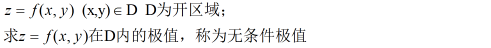无条件极值的判别步骤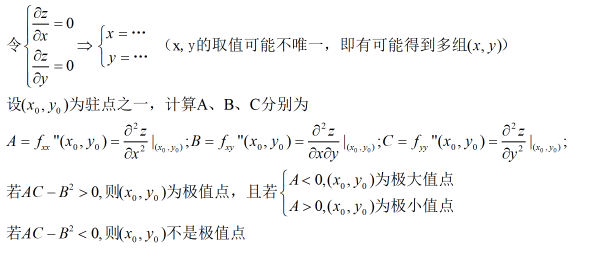例题
例1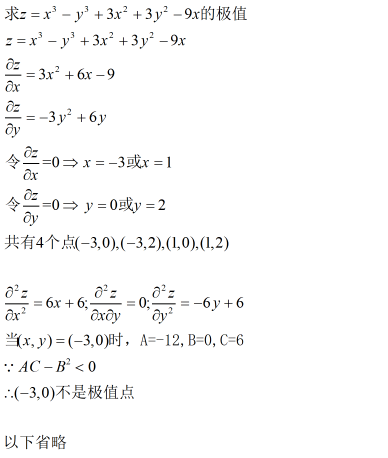条件极值
Case 1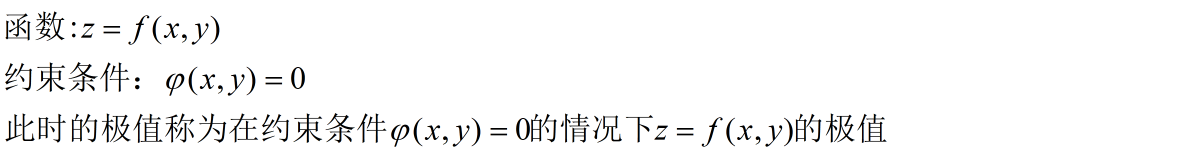求条件极值的步骤：
第一步：使用拉格朗日乘除法构造函数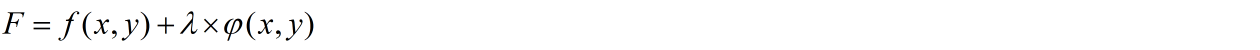第二步，求三个偏导数，令之等于0，解出x,y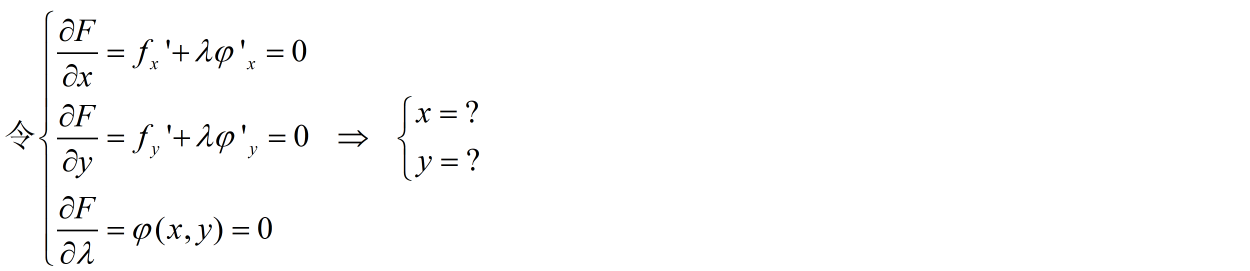解出x,y后可能会得到不止一组点，此时，将之代入，最大的就是最大值，最小的就是最小值，反正就是全部代进去。
例题
例2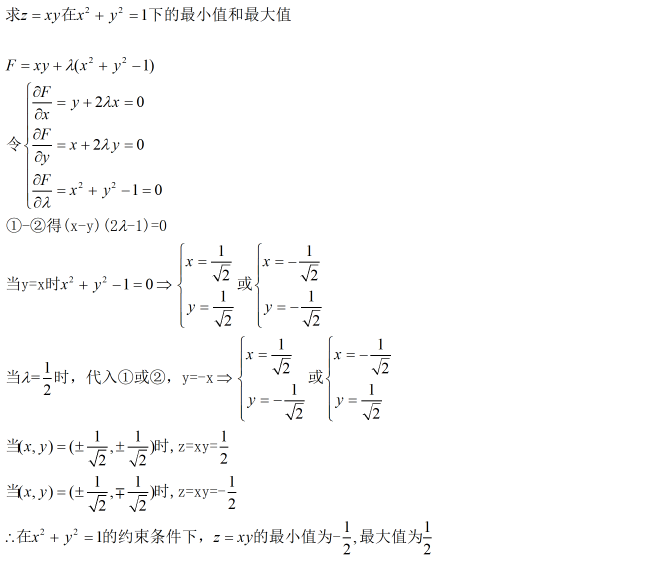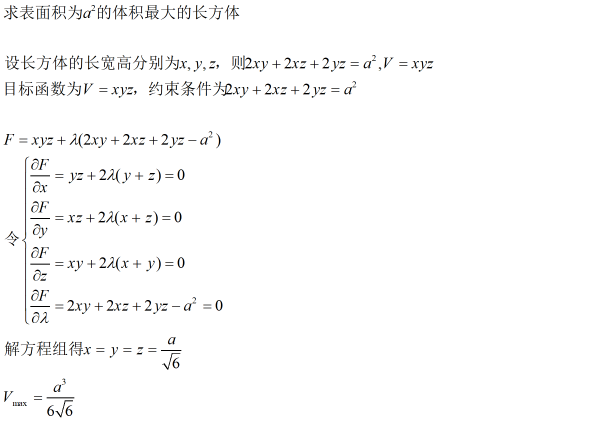Case 2
条件极值的第二种形式，约束条件是一个方程组步骤类似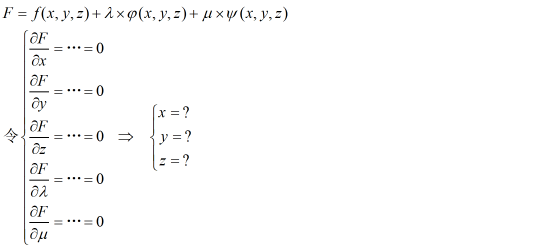以上就是本篇的全部内容，无条件极值的情况要算ABC判别式，条件极值就是构造方程然后解方程组就行了。
本篇完。


展开全文• 多元函数极值条件 ppt 最优化 机器学习 数值分析最优化
• 多元函数的基本概念 解释全面 思路清晰，内容完整，原创！ 最完整的，最易懂的 PPT
• 一元函数，多元函数，可微的含义：就是用极限的思想近似反应两个可变因素之间的函数关系。近似代替。 一元函数微分的几何意义：就是曲线x增加了一部分，y增加多少的表示。用了极限分割的思想，无线接近。dy与△y...
• 多元函数极值与AAA及Δ=B2−4AC\Delta =B^2-4ACΔ=B2−4AC的关系
• 多元函数极值问题 可以分为以下三个方面 无约束极值问题 等式约束条件极值问题 不等式约束条件极值问题 无约束条件的多元函数极值算法
• 多元函数极值问题的分析与研究，郭常予，徐玲，本文主要讨论Hessian 判别法失效情况下， 如何判定多元数值函数（特别是二元函数）极值问题。首先，简要介绍了多元函数极值问题对�
• 今天小编就为大家分享一篇TensorFlow 多元函数的极值实例，具有很好的参考价值，希望对大家有所帮助。一起跟随小编过来看看吧
• 多元函数的微分 导数概念的形式化 一、总论 ​ 数值多元函数微分及其相关概念，如中值定理、泰勒展开是数值一元函数的一般化。更进一步说，最一般化的东西是向量值函数。我写这一节的目的是为了与书中特殊到一般的...
• ## Hessian矩阵与多元函数极值

万次阅读 多人点赞 2016-04-17 14:13:50
海塞矩阵（Hessian Matrix）是一个多元函数的二阶偏导数构成的方阵。它在机器学习和图像处理中，具有重要应用。例如，图像特征检测中的SIFT和SURF算法中就用到了它。此外，很多机器学习算法（例如Logistics回归）的...机器学习 图像处理 数学 海塞矩阵
• 个人重点： 1.二元函数的无条件极值的充分条件 2.二元函数的条件极值的必要条件— 拉格朗日数乘法 可微定义判别法变形也...多元函数的最大最小值问题 海伦公式 均值不等式 ...python qt 机器学习
• §8.8 多元函数极值及其求法 一、多元函数的极值 1、多元函数极值定义 设函数在点的某个邻域内有定义,对该邻域内异于的点,如果都适合不等式 则称函数在点取极大值； 如果都适合不等式 则称函数在点取极小...
• 本节为高等数学复习笔记的第七部分，多元函数微分学（2），主要包括：多元微分法求多元偏导数 。 2. 多元函数极值 2.1 二元函数取极值的必要条件（适用于多元）   设z=f(x,y)在点(x0,y0)处一阶偏导数设z=f(x,y)在...高等数学
•期末复习
•学习笔记
• 高等数学教材中，多元函数微分法及其应用讲义。共分为8节。包含隐函数求导，方向导数与梯度，偏导数，多元函数极值求法等...